Example Questions

← Previous 1

Example Question #1 : Factoring Polynomials

Which of the following is a factor of the polynomial 3y2+14y-24?

3y+4

y-6

y+6

4y-3

3y-6

y+6

Explanation:

The polynomial factors to (3y-4)(y+6).  (y+6) is the only one of those two options available as an answer.

Example Question #71 : Polynomials

Factor the polynomial : x3 + 27

(x +3) (x2 - 3x +9)

(x2 + 3) (x – 3x +9)

(x +3) (x2 - 3x +3)

(x +9) (x2 - 3x +9)

(x +3) (x2 - 3x +9)

Explanation:

First, write as a sum of the cubes,

x3 + 33,  then factor : (x + 3) (x2 – 3x + 32).  Apply the exponent:

(x + 3) (x2 – 3x + 9)

Example Question #71 : Polynomials

Factor the polynomial: 2x2 + ab – 2b – ax2

(x2 – 2) (a – b)

(b - X2) (a + 2)

(x2 + b) (a - 2)

(x2 – b) (2 - a)

(x2 – b) (2 - a)

Explanation:

2x2 + ab – 2b – ax2 = 2x2 – 2b – ax2 + ab

Rearrange  terms

= (2x2 – 2b) + (- ax2 + ab)   Group

= 2(x2 – b) + a(-x2 + b)        Factor each group

= 2(x2 – b) – a(x2 – b)          Factor out  -a

= (x2 – b) (2 – a)                  Factor out x2 – b

Example Question #1 : Factoring Polynomials

Which of the following is a factor of the polynomial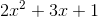?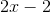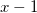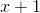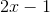Explanation:

Factor the polynomial by choosing two values that when FOILed will sum to the middle coefficient, 3, and multiply to 2.  These two numbers are 1 and 2.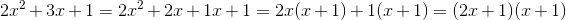Only (x +1) is one of the choices listed.

Example Question #5 : Factoring Polynomials

Which of the following expressions is a factor of this polynomial?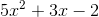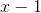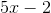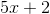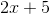Explanation:

The polynomial factors into the following expression: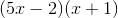Therefore, the answer isExample Question #6 : Factoring Polynomials

Which of the following is equivalent to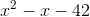?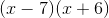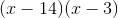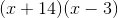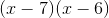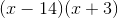Explanation:

You have two options for a problem like this. On the one hand, you can merely FOIL the answers until you find one that equals the value given in the equation. The other option is to factor it adequately from the beginning. This is not too hard. Since the middle term is so small, you know that the numeric portions of your factors will have to be nearly equal. Since we know that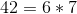, this is very easy! Given that the last value is a negative number, you know that your two groups need to be a combination of addition and subtraction.

Thus, your answer is:Example Question #7 : Factoring Polynomials

Which of the following is equivalent to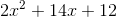?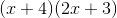This cannot be factored.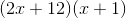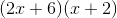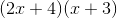Explanation:

You have two options for a problem like this. On the one hand, you can merely FOIL the answers until you find one that equals the value given in the equation. The other option is to factor it adequately from the beginning. For a question like this, it is a bit harder, given that there is a number in front of the first term. Now, given the signs in the original problem, you know that your groups will look like the following: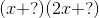Now, you can do a little trick to make your life easier. Factor out the common: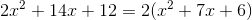The latter is very easy to factor: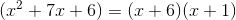Now, you have: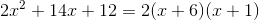You can distribute theinto either group. For the answers given, the only correct value is:Example Question #8 : Factoring Polynomials

Which of the following is equivalent to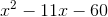?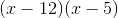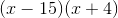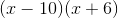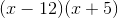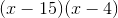Explanation:

You have two options for a problem like this. On the one hand, you can merely FOIL the answers until you find one that equals the value given in the equation. The other option is to factor it adequately from the beginning. This is not too hard. You know that your groups will need to have one positive and one negative between them (this is because of the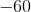at the end of your original polynomial). Now, you need to carefully pick your factors. It certainly is notand; however, neither willandnorandwork. Notice thatanddoes the trick:Example Question #9 : Factoring Polynomials

Factor the following polynomial: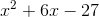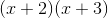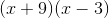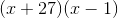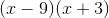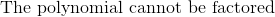Explanation:

To factor the polynomial, factor the last term into two numbers that sum to the middle term.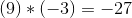and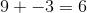so we simply place those into parentheses to obtain:Example Question #10 : Factoring Polynomials

The polynomial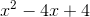is equal to which of the following expressions.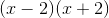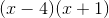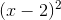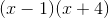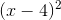Explanation:

This question calls for us to factor the polynomial into two binomials.

Since the first term isand the last term is a number without a variable, we know that how answer will be of the form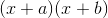where a and b are positive or negative numbers.

To find a and b we look at the second and third term. Since the second term is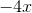we know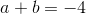. (The x comes from a and b multiplying by x and then adding with each other). The +4 term tells us that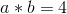. Using these two pieces of information we can look at possible values. The third term tells us that 1 & 4, 2 & 2, -1 & -4, and -2 & -2 are the possible pairs.

Now we can look and see which one adds up to make -4.

This gives us the pair -2 & -2.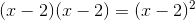← Previous 1